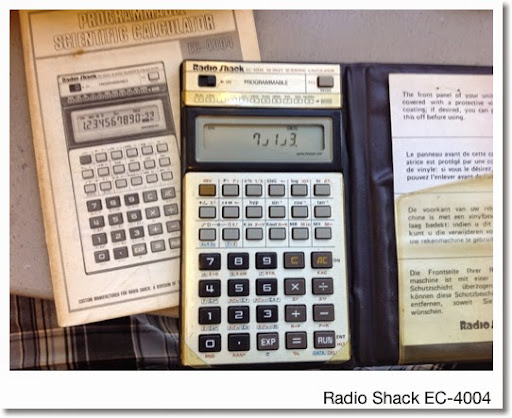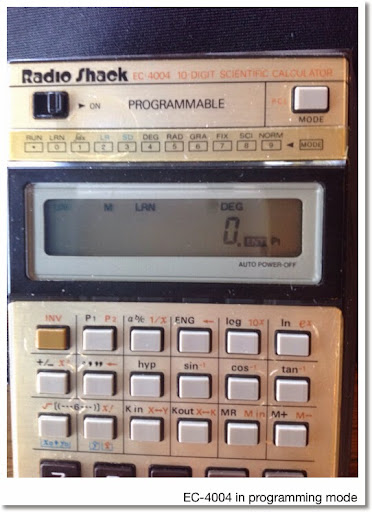## Sunday, September 14, 2014I recently purchased two calculators from https://www.devicegoround.com:

The EC-4004 is similar to the Casio fx-3600P, both manufactured beginning in 1981. The EC-4004 is battery powered taking two smaller-sized button batteries. (Currently it is running on LR-1130 and Radio Shack carries equivalent sized batteries.)

In addition to the independent memory (M), the EC-4004 has six additional memory registers. The Kin key stores the number into one of the six registers, which the Kout key is the recall key. In spite of its manual not mentioning this, the EC-4004 supports storage arithmetic.

Storage Arithmetic:

Let # represents registers 1 through 6:

Add x to #: x [ Kin ] [ + ] #
Subtract x from #: x [ Kin ] [ - ] #
Multiply x to #: x [ Kin ] [ × ] #
Divide #/x: x [ Kin ] [ ÷ ] #

Features:

* Single Variable Statistics and Linear Regression

* Programming including integrals

* Fractions. Kind of limited, you can enter fractions in operations and get answers in fractions (simplest form). Once a number in decimal form is entered, the result will be in decimal form. No converting from fractions to decimal feature is present, either.

* ENG and <-ENG key. These represent a number in different ways:

ENG: decreases the exponent part by 10^3, multiplies the mantissa by 1000
<-ENG: increases the exponent part by 10^3, divides the mantissa by 1000

Example: 23400 [ = ]
[ ENG ] (23.4 x 10^3)
[ ENG ] (23400 x 10^0)
[ ENG ] (23400000 x 10^-3)

23400 [ = ]
[ <-ENG ] (0.0234 x 10^6)
[ <-ENG ] (0.0000234 x 10^9)
[ <-ENG ] (0.0000000234 x 10^12)

Operating System

The operating system is AOS (Algebraic Operating System). I realize that AOS is a Texas Instruments term, however, it applies here. Simply put, any one-argument function (e^x, trig, log) are pressed after the number is entered. Example:

ln 2431.74 is calculated as 2431.74 [ ln ] (Result: 7.796362329)

Programming

Learn Mode: Mode 0, press [ P1 ] or [ INV ] [ P1 ] for P2.
Run Mode: Mode Decimal Point ( . ). Execute programs by pressing [ P1 ] or [ INV ] [ P1 ] for P2.

Memory: 38 steps, fully-merged
Number of Program Slots: 2

Not a lot of room, but it is handy for storing quick calculations. You have to be real precise in entering keystrokes because there are no editing features. We do have some tools in programming mode:

[ RUN ] (ENT): Acts as a stop, which will prompt the user to enter numbers. A program stopped midway has an ENT indicator. In run mode, the [ RUN ] continues program execution.

You can use [ RUN ] in programming mode prior to entering number (acts a placeholder). The number itself will not be stored as a step. This is important as programs may not run correctly without this step. Make sure that the placeholder numbers used make the calculations valid for each step!

x > 0 ( [ INV ] [ 7 ] ): Tests the number in the display. If the number is greater than zero, control goes back to the beginning. Execution stops.

x ≤ M ( [ INV ] [ 8 ] ): Tests whether the number in the display is equal to or less than what is stored in the independent Memory M. If the test is true, return to the beginning of the program. Execution stops.

RTN ( [ INV ] [ 9 ] ): Stops execution and returns to the beginning of the program.

[ INV ] [ MODE ]: is the clear program command. This is extremely important to do before starting any new problems.

Mode 1 is the Integral mode. Use P1 or P2 as the function. According to the manual, start going into Learn mode. Begin the program by pressing (number if needed) [ INV ] [ MR ] (Min). Use [ MR ] for the independent variable. End the function with [ = ]. Go into Mode 1, press P1 or P2, then lower limit, [ RUN ], upper limit, [ RUN ]. The EC-4004 stores the following in its registers:

1: lower limit
2: upper limit
3: subdivisions (store by INV RUN)
4: f(lower limit)
5: f(upper limit)
6: numeric integral

Sample Programs

Area of an Ellipse

A = π * a * b

Mode 0, P1: I am using 1 as placeholders.
ENT ( [ RUN ] key) 1
[ × ]
ENT 1
[ × ]
[EXP] key for π
[ = ]
RTN

Run P1: enter a, press [ RUN ], enter b, press [ RUN ], get the area.
Press [ AC ] to leave execution mode.

Examples:
a = 6, b = 3, Area = 56.54866776
a = 3, b = 8.1, Area = 76.340707148

Discriminant Tester

Here is a program where the placeholder numbers are important - all steps must count as valid.

Program: Calculate b^2 - 4ac. If positive, calculate its square root. If not, return to the beginning of the program and display 0.

Use the x ≤ M test.

I used P2 for this example:
0
[ INV ] [ MR ] (Min)
ENT 8
[ INV ] [ +/- ] x^2
[ - ]
4
[ × ]
ENT 3
[ × ]
ENT -2
[ = ]
[ INV ] [ RUN ] (HLT)
[ INV ] [ 8 ] x≤M (x ≤ 0?)
[ INV ] [ ( ] ( √ )

Note the order of entry: b, a, c

Examples:
b = 8, a = 3, c = -2. Results: 88, 9.38083152.
b = 1, a = 1, c = 7. Results: -27, 0. Press [ AC ]

Integral: an example

Calculate ∫ x * e^x dx from x =1 to x = 2. Use P1.

Keystrokes:

Enter the function:
[ MODE ] [ 0 ] (LRN)
[ P1 ]
[ INV ] [ MR ] (Min)
[ MR ]
[ × ]
[ MR ]
[ INV ] [ ln ] (e^x)
[ = ]

Enter integration mode:
[ MODE ] [ 1 ] ( ∫dx is displayed)
[ P1 ]
1 [ RUN ] 2 [ RUN ]

Approximate Result: 7.38906 x 10^0 (7.38906)

Source: "Programmable EC-4004 Scientific Calculator Owner's Manual" Radio Shack, 1981.

This may be my last blog entry before I head to Reno, NV, next weekend. I am going to the HHUC (HP Handhelds User Community) 2014 conference, http://hhuc.us/2014/index.htm . I plan to blog about some of the highlights of the conference in a future blog post. Until then, take care and thanks for your subscriptions, comments, and questions.

Eddie

This blog is property of Edward Shore. 2014### HP 15C: Error Function and Lower Tail Normal Cumulative Function

HP 15C:   Error Function and Lower Tail Normal Cumulative Function Formulas Used  Error Function  erf(x) = 2 ÷ √π * ∫( e^(-t^2) dt, t = 0 t...